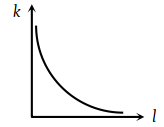You’ve reached the end of your free Videos limit.
#7 | Mechanical Energy
(Physics) > Work,Energy and Power
Related Practice Questions :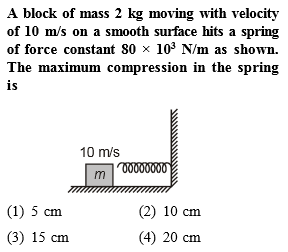Complete Question Bank + Test Series
Complete Question Bank

Difficulty Level: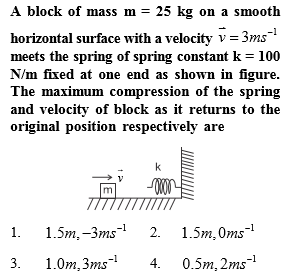Complete Question Bank + Test Series
Complete Question Bank

Difficulty Level:

A mass m slips along the wall of a semispherical surface of radius R. The velocity at the bottom of the surface is [ MP PMT 1993]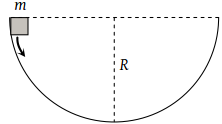(1) $\sqrt{Rg}$

(2) $\sqrt{2Rg}$

(3) $2\sqrt{\pi Rg}$

(4) $\sqrt{\pi Rg}$

Complete Question Bank + Test Series
Complete Question Bank

Difficulty Level:

Three different objects of masses and m3 are allowed to fall from rest and from the same point ‘O’ along three different frictionless paths. The speeds of the three objects, on reaching the ground, will be in the ratio of

(1) ${m}_{1}:{m}_{2}:{m}_{3}$

(2) ${m}_{1}:2{m}_{2}:3{m}_{3}$

(3) 1 : 1 : 1

(4) $\frac{1}{{m}_{1}}:\frac{1}{{m}_{2}}:\frac{1}{{m}_{3}}$

Complete Question Bank + Test Series
Complete Question Bank

Difficulty Level:

Which of the following graph depicts spring constant k versus length l of the spring correctly

 (1)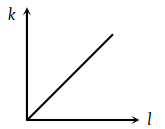(2)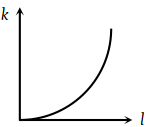(3)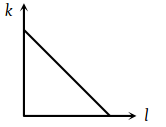(4)